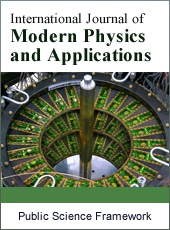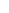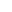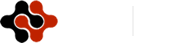International Journal of Modern Physics and Applications
Articles Information
International Journal of Modern Physics and Applications, Vol.5, No.2, Jun. 2019, Pub. Date: Jun. 15, 2019
Approximate Solution of Single Spring Moving System for Damped and Undamped Simple Harmonic Motion by Using Homotopy Perturbation Method
 Mohammad Masud Rana, Department of Mathematics, Faculty of Science, Islamic University, Kushtia, Bangladesh.
 Ahammodullah Hasan, Department of Mathematics, Faculty of Science, Islamic University, Kushtia, Bangladesh.
In this paper, Homotopy perturbation method (HPM) is applied to find the approximate solution of simple harmonic motion of single spring equation, which is known a well-known nonlinear ordinary differential equation. The Homotopy Perturbation Method deforms a difficult problem into a simple problem which can be easily solved. Firstly, the approximate solution of single spring equation is developed using initial conditions. Then the results are compared with the results obtained by Numerical solutions. Finally, Homotopy Perturbation Method (HPM) is applied to find the approximate solution of single spring equation with initial conditions. The results reveal that the HPM is very effective, convenient and quite accurate to systems of nonlinear equations. Some examples are presented to show the ability of the method for moving single spring equation.
Homotopy Perturbation Method (HPM), Simple Harmonic Motion, Nonlinear Differential Equation, Damped, Undamped, Approximate Solution, Numerical Solution
 A. Ghorbani and J. S. Nadjafi, “He’s homotopy perturbation method for calculating Adomian’s polynomials,” Int. J. Nonlin. Sci. Numer. Simul., 8, No. 2, 229–332 (2007); https://doi.org/10.1515/IJNSNS.2007.8.2.229
 J. H. He, “Application of homotopy perturbation method to nonlinear wave equations” Journal of Chaos, Solitons & Fractals, Volume 26, Issue 3, November 2005, Pages 695-700; https://doi.org/10.1016/j.chaos.2005.03.006
 J. H. He,“Some asymptotic method for strongly”, International Journal of Modern Physics, Vol. 20, No. 10 (2006); 1141–1199; https://www.researchgate.net/publication/228644456
 M. A. Noor and S. T. Mohyud-Din, “ Modified variational iteration method for solving Fisher’s equations”, Journal of Applied Mathematics and Computing, Volume 31, pp. 295–308, (2009), https://link.springer.com/article/10.1007/s12190-008-0212-7
 M. A. Noor and S. T. Mohyud-Din, “Homotopy perturbation method for solving sixth-order boundary value problems”, Computers & Mathematics with Applications Volume 55, pp. 2953-2972, (2008) https://doi.org/10.1016/j.camwa.2007.11.026
 M. A. Haji and K. Al-Khaled, “Analytic studies and numerical simulations of the generalized Boussinesq equation”, Applied Mathematics and Computation, Vol. 191,, PP. 320-333, (2007), https://doi.org/10.1016/j.amc.2007.02.090
 M. A Noor, S. T. Mohyud-Din, “ Homotopy Perturbation Method and Pade Approximants for Solving Flierl-Petviashivili Equation”, Applications and Applied Mathematics: An International Journal, Vol. 3, No. 2, PP. 224-234, (2008), https://www.pvamu.edu/aam/
 J. H. He., “Homotopy perturbation technique”, Computer Methods in Applied Mechanics and Engineering, Vol. 178, Pp. 257-262, (1999), https://doi.org/10.1016/S0045-7825(99)00018-3
 J. H. He., (2000a), “A coupling method of Homotopy technique and a perturbation technique for nonlinear problems”, International Journal of Nonlinear Mechanics, 35, pp. 37-43.
 J. H. He., (1999), “Homotopy perturbation technique computational methods”, Applied Mechanics and Engineering 178, pp. 257-262.
 J. H. He., (2000a), “A coupling method of homotopy technique and a perturbation technique for nonlinear problems”, International Journal of Nonlinear Mechanics 35, pp. 37-43.
 J. H. He., (2003a), “A simple perturbation approach to Blasius equation”, Applied Mathematics and Computation 140, pp. 217-222.
 J. H. He., (2003b), “Homotopy Perturbation Method: A new nonlinear analytical technique”, Applied Mathematics and Computation 135, pp. 73-79.
 J. H. He., (2005), “Application of homotopy perturbation method to nonlinear wave equation”, Chaos Solutions Fractals 26, pp. 295-300.
 J. H. He., (2006). Homotopy perturbation method for solving boundary value problem. Physics Letters A 350, pp. 87-96.
 Ganji DD and Rafei M (2006), “Solitary wave solutions for a generalisezed Hirota- Satsuma coupled Kdv equations by homotopy perturbation method”, Physics Letters A 356, pp. 131-137.
 Biazer JH and Ghazvini (2009), “He’s homotopy perturbation method for solving systems of volterra integral equations”, Chaos Solitons and Fractals 39, pp. 370-377.
 Rafiq A, Hussain S, and Ahmed M (2009), “General homotopy method for Lane-Emden type differrential equations”, International Journal of Applied Mathematics and Mechanics, 5 (3), pp. 75-83.
 Abbasbandy S (2007), “Application of He’s homotopy perturbation method to functional integral equations”, Chasos Solitons and Fractals 31, pp. 1243-1247.
 Wazwaz AM (2004), “The tanh method for travelling wave solutions of nonlinear equations”, Applied Mathematics and Computation, 154, pp. 713–723.ISSN Print: 2381-6945
ISSN Online: 2381-6953
Current Issue:
Vol. 5, Issue 3, September Submit a Manuscript Join Editorial Board Join Reviewer Team
 About This Journal All Issues Open Access Indexing Payment Information Author Guidelines Review Process Publication Ethics Editorial Board Peer Reviewers600 ATLANTIC AVE, BOSTON,
MA 02210, USA+001-6179630233
JournalsJournal ListsAuthor GuidelinesPayment InformationSubmit a ManuscriptJoin as Editorial MembersJoin as ReviewersPrivacy NoticeTerms of ConditionsHelp Center